# Steady State AC Magnetic : solved equations (vector potential 3D)

## Introduction

The magnetic vector potential is a proposed model for 3D applications.

## Equation solved with magnetic vector potential model (3D)

The equation solved by the finite elements method in a Steady State AC

Magnetic application is written: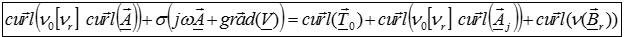Where :

r] is the tensor of the reluctivity of the medium (in m/H)

ν0 is the magnetic reluctivity of the vacuum ; ν0 = 1/μ0 = 1/(4 π 10-7) (en m/H)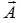is the magnetic vector potential (in Wb/m)

σ is the electrical conductivity of the medium (in S)

J is the complexe number as J2 = -1

ω is the pulsation (in rd/s)

V is the magnetic scalar potential (in A/m²)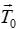is the source electric vector potential source (in A/m)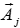is the source magnetic vector potential (in Wb/m)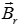is the induction of the magnets (in Wb/m)

## Formulations in Flux

Problems

Formulations (in Flux 3D)

Solid conductors with unmeshed coils coupled circuit or not and meshed coils coupled circuits or not. AWVT0WAJW
Other regions AWT0WAJW